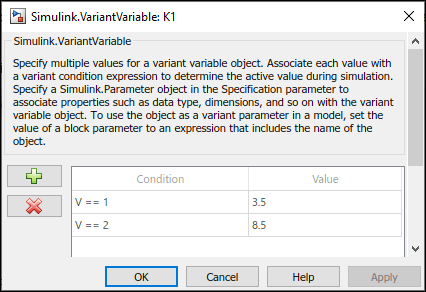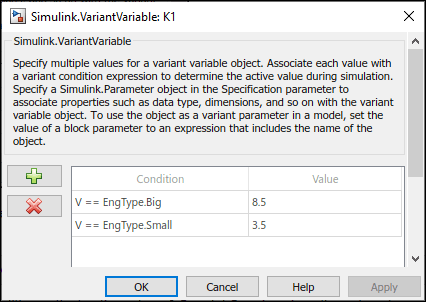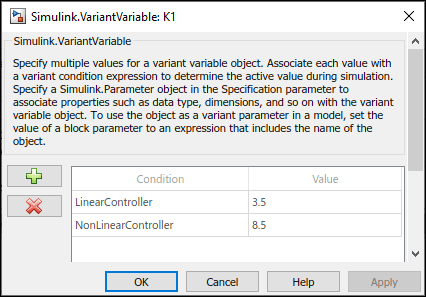## Use Variant Control Variables in Variant Parameters

This topic explains how to use different types of variant control variables in variant parameters.

Consider the `slexVariantParameters` model.The Gain parameter of the Gain blocks are variant parameters that are specified as `Simulink.VariantVariable` objects, `K1` and `K2`. `K1` and `K2` have multiple values associated with variant condition expressions `V == 1` and `V == 2`. Here, the variant control variable `V` that determines the active value of `K1` and `K2` is a `Simulink.VariantControl` object. You can change `V` to any of these types based on your requirement.

Note

Before you start with these examples, we recommend you complete Create a Simple Variant Parameter Model.

### Numeric Variant Control Values for Rapid Prototyping of Variant Parameters

Numeric values allow you to rapidly prototype variant values when you are still building your model. Numeric values help you focus more on building your variant values than on developing the expressions that activate those choices.

You can define numeric control values in locations listed in Storage Locations for Variant Control Variables (Operands) in Variant Blocks.

Open the `slexVariantParameters` model.

`open_system('slexVariantParameters')`

In the MATLAB Editor, specify variant choices in their simplest form as numeric values in `Simulink.VariantVariable` objects `K1` and `K2`.

`K1 = Simulink.VariantVariable('Choices',{'V==1', 3.5, 'V==2', 8.5})`
```K1 = VariantVariable with 2 choices: Condition Value _________ _____ V == 1 3.5000 V == 2 8.5000 Specification: '' Use getChoice, setChoice, addChoice, removeChoice to access, modify, add or remove choices ```
`K2 = Simulink.VariantVariable('Choices',{'V==1', 4.5, 'V==2', 9.5})`
```K2 = VariantVariable with 2 choices: Condition Value _________ _____ V == 1 4.5000 V == 2 9.5000 Specification: '' Use getChoice, setChoice, addChoice, removeChoice to access, modify, add or remove choices ```

Once you successfully create the `Simulink.VariantVariable` objects, you can modify them by using the methods described in Public Methods or from the `VariantVariable` dialog box.Activate one of the variant values by defining a control variable, `V`, and setting its value to `1` in a `Simulink.VariantControl` object.

`V = Simulink.VariantControl('Value', 1, 'ActivationTime', 'update diagram')`
```V = VariantControl with properties: Value: 1 ActivationTime: 'update diagram' ```

When you simulate the model, the condition `V == 1` evaluates to `true`. `K1 ` is assigned a value of `3.5 `and `K2 `is assigned a value of` 8.5`.

`sim ('slexVariantParameters')`

If you change the value of `V` to `2`, Simulink® sets the value of `K1` and `K2 `to `4.5` and `9.5` during simulation. You can change the value of `V` using this command or from `Simulink.VariantControl` dialog box.

```V.Value = 2; sim ('slexVariantParameters')```

### Simulink.Parameter Type of Variant Control Variables for Code Generation of Variant Parameters

If you intend to generate code for a model containing variant parameters, specify variant control variables as `Simulink.Parameter` objects. `Simulink.Parameter` objects allow you to specify other attributes, such as data type and storage class, and control the appearance and placement of variant control variables in generated code.

• You can define a variant control variable of type `Simulink.Parameter` only in the base workspace or in a data dictionary. Defining `Simulink.Parameter` type of variant control variables in the mask or model workspace is not supported. For more information on storage locations for variant control variables, see Storage Locations for Variant Control Variables (Operands) in Variant Blocks.

• `Simulink.Parameter` objects within structures and that have data types other than `Simulink.Bus` objects are not supported.

Open the `slexVariantParameters` model.

`open_system('slexVariantParameters')`

In the MATLAB Editor, define a `Simulink.Parameter` object.

```VSS_MODE = Simulink.Parameter; VSS_MODE.Value = 1; VSS_MODE.DataType = 'int32'; VSS_MODE.CoderInfo.StorageClass = 'Custom'; VSS_MODE.CoderInfo.CustomStorageClass = 'Define'; VSS_MODE.CoderInfo.CustomAttributes.HeaderFile ='demo_macros.h'; ```

Variant control variables defined as `Simulink.Parameter` objects can have any of the storage classes listed in Storage Classes for Different Variant Activation Times (Embedded Coder).

You can also convert a scalar variant control variable into a `Simulink.Parameter` object. For more information, see Convert Variant Control Variables into Simulink.Parameter Objects.

Specify the object as a variant control in `Simulink.VariantVariable` objects `K1` and `K2`.

`K1 = Simulink.VariantVariable('Choices',{'V == 1', 3.5, 'V == 2', 8.5})`
```K1 = VariantVariable with 2 choices: Condition Value _________ _____ V == 1 3.5000 V == 2 8.5000 Specification: '' Use getChoice, setChoice, addChoice, removeChoice to access, modify, add or remove choices ```
`K2 = Simulink.VariantVariable('Choices',{'V == 1', 4.5, 'V == 2', 9.5})`
```K2 = VariantVariable with 2 choices: Condition Value _________ _____ V == 1 4.5000 V == 2 9.5000 Specification: '' Use getChoice, setChoice, addChoice, removeChoice to access, modify, add or remove choices ```

Once you successfully create the `Simulink.VariantVariable` objects, you can modify them by using the methods described in Public Methods or from the `VariantVariable` dialog box.Activate one of the variant values by defining a control variable `V` and setting its value to `VSS_MODE` in a `Simulink.VariantControl` object.

`V = Simulink.VariantControl('Value',VSS_MODE,'ActivationTime','code compile')`
```V = VariantControl with properties: Value: [1x1 Simulink.Parameter] ActivationTime: 'code compile' ```

When you simulate the model, the condition `V == 1` evaluates to `true`. `K1` is assigned a value of `3.5`, and `K2` is assigned a value of `8.5`.

`sim ('slexVariantParameters')`

If you change the value of `VSS_MODE` to `2`, Simulink® sets the value of `K1` and `K2` to `4.5` and `9.5` during simulation. You can change the value of `V` using this command or from `Simulink.VariantControl` dialog box.

```VSS_MODE.Value = 2; V.Value = VSS_MODE; sim ('slexVariantParameters')```

Generate code from the model. For information on how to generate code, see Generate Code Using Embedded Coder (Embedded Coder)

The generated code contains both `Linear` and `Nonlinear` choices in preprocessor conditionals `#if` and `#elif` because of the `code compile` activation time. The variant control variable `V` is defined using a macro — `#define` directive — in the header file `demo_macros.h`. You can control the appearance and placement of `V` in the generated code and prevent optimizations from eliminating storage for `V` using the storage class property. For more information, see Storage Classes for Different Variant Activation Times (Embedded Coder).

```% demo_macros.h % /* Exported data define */ % % /* Definition for custom storage class: Define */ % #define V 2 /* Referenced by: % * '<Root>/Gain' % * '<Root>/Gain1' % */ % #endif /* RTW_HEADER_demo_macros_h_ */ % % /*```

### Enumerated Types to Improve Code Readability of Variant Control Variables of Variant Parameters

Use enumerated types to give meaningful names to integers used as variant control values.For more information on enumerated type data, see Use Enumerated Data in Simulink Models.

In the MATLAB® Editor, define the classes that map enumerated values to meaningful names.

Open the `slexVariantParameters` model.

`open_system('slexVariantParameters')`

Specify the variant condition expressions `V == EngType.Small` and `V == EngType.Big` in `Simulink.VariantVariable` objects `K1` and `K2`.

`K1 = Simulink.VariantVariable('Choices', {'V == EngType.Small',3.5,'V == EngType.Big',8.5})`
```K1 = VariantVariable with 2 choices: Condition Value __________________ _____ V == EngType.Big 8.5000 V == EngType.Small 3.5000 Specification: '' Use getChoice, setChoice, addChoice, removeChoice to access, modify, add or remove choices ```
`K2 = Simulink.VariantVariable('Choices', {'V == EngType.Small',4.5,'V == EngType.Big',9.5})`
```K2 = VariantVariable with 2 choices: Condition Value __________________ _____ V == EngType.Big 9.5000 V == EngType.Small 4.5000 Specification: '' Use getChoice, setChoice, addChoice, removeChoice to access, modify, add or remove choices ```

Here, `EngType` is an integer-based enumeration class that is derived from the built-in data type, `int32`. The class has two enumeration values, `Small` and `Big`. These enumerated values have underlying integer values `1` and `2`. In this example, the enumeration class is defined in the base workspace. You can choose to define the class in other storage locations as listed in Storage Locations for Variant Control Variables (Operands) in Variant Blocks.

`type EngType.m`
```classdef EngType < Simulink.IntEnumType enumeration Small (1) Big (2) end end ```

Once you successfully create the `Simulink.VariantVariable` objects, you can modify them by using the methods described in Public Methods or from the `VariantVariable` dialog box.Activate one of the variant values by defining the control variable `V` and setting its value to `EngType.Small` in `Simulink.VariantControl` object `V`.

`V = Simulink.VariantControl('Value',EngType.Small,'ActivationTime','code compile')`
```V = VariantControl with properties: Value: Small ActivationTime: 'code compile' ```

When you simulate the model, the condition `V == 1` evaluates to `true`. `K1` is assigned a value of `3.5`, and `K2` is assigned a value of `8.5`.

`sim('slexVariantParameters')`

If you change the value of `V` to `2`, Simulink® sets the value of `K1` and `K2` to `4.5` and `9.5` during simulation. You can change the value of `V` using this command or from `Simulink.VariantControl` dialog box.

`V.Value = 2`
```V = VariantControl with properties: Value: 2 ActivationTime: 'code compile' ```
`sim ('slexVariantParameters')`

The code that you generate using enumerated types contains the names of the values rather than integers.

```% slexVariantParameters_private.h % #if V == EngType_Big || V == EngType_Small % /* Variable: K1 Referenced by: '<Root>/Gain' */ % #if V == EngType_Big % #define rtCP_Gain_K1 (8.5) % #elif V == EngType_Small % #define rtCP_Gain_K1 (3.5) % #endif % #endif % % #if V == EngType_Big || V == EngType_Small % /* Variable: K2 Referenced by: '<Root>/Gain1' */ % #if V == EngType_Big % #define rtCP_Gain1_K2 (9.5) % #elif V == EngType_Small % #define rtCP_Gain1_K2 (4.5) % #endif % #endif % #endif /* RTW_HEADER_slexVariantParameters_private_h_ */```

Note that for variant parameters with startup activation time, only enumerations that are defined using these techniques are supported:

• Using the function `Simulink.defineIntEnumType`

• By subclassing built-in integer data types `int8`, `int16`, `int32`, `uint8`, or `uint16`, or by subclassing `Simulink.IntEnumType`

These enumerations are also supported when permanently stored in a Simulink® data dictionary. See Enumerations in Data Dictionary.

### Simulink.Variant Objects for Variant Condition Reuse of Variant Parameters

After identifying the variant values that your model requires, you can construct complex variant conditions to control the activation of your variant parameter values by defining variant conditions as `Simulink.Variant` objects. `Simulink.Variant` objects enable you to reuse common variant conditions across models and help you encapsulate complex variant condition expressions.

• You can define a variant control variable of type `Simulink.Variant` only in the base workspace or in a data dictionary. Defining `Simulink.Variant` type of variant control variables in the mask or model workspace is not supported. For more information on storage locations for variant control variables, see Storage Locations for Variant Control Variables (Operands) in Variant Blocks.

• `Simulink.Variant` within structures are not supported.

Open the `slexVariantParameters` model.

`open_system('slexVariantParameters')`

In the MATLAB® Editor, encapsulate variant control expressions as `Simulink.Variant` objects.

```LinearController=Simulink.Variant('V==1'); NonLinearController=Simulink.Variant('V==2');```

Specify the `Simulink.Variant` objects as the variant controls in a `Simulink.VariantVariable` objects `K1` and `K2`.

`K1 = Simulink.VariantVariable('Choices',{'LinearController',3.5,'NonLinearController',8.5})`
```K1 = VariantVariable with 2 choices: Condition Value ___________________ _____ LinearController 3.5000 NonLinearController 8.5000 Specification: '' Use getChoice, setChoice, addChoice, removeChoice to access, modify, add or remove choices ```
`K2 = Simulink.VariantVariable('Choices',{'LinearController',4.5,'NonLinearController',9.5})`
```K2 = VariantVariable with 2 choices: Condition Value ___________________ _____ LinearController 4.5000 NonLinearController 9.5000 Specification: '' Use getChoice, setChoice, addChoice, removeChoice to access, modify, add or remove choices ```

Once you successfully create the `Simulink.VariantVariable` objects, you can modify them by using the methods described in Public Methods or from the `VariantVariable` dialog box.Activate one of the variant values by defining a `Simulink.VariantControl` object `V` and setting its value to `1`.

`V = Simulink.VariantControl('Value',1,'ActivationTime','update diagram')`
```V = VariantControl with properties: Value: 1 ActivationTime: 'update diagram' ```

When you simulate the model, the condition `V == 1` evaluates to `true`. `K1` is assigned a value of `3.5`, and `K2` is assigned a value of `8.5`.

`sim('slexVariantParameters')`

If you change the value of `V` to `2`, Simulink® sets the value of `K1` and `K2` to `4.5` and `9.5` during simulation. You can change the value of `V` using this command or from `Simulink.VariantControl` dialog box.

```V.Value = 2; sim ('slexVariantParameters')```

Using this approach, you can develop complex variant condition expressions that are reusable.